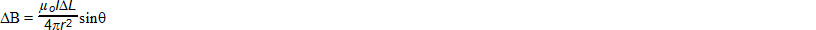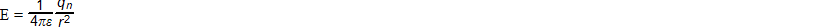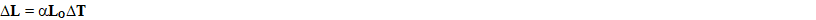### AP Physics 1 Week 10 (Aug 13 - Aug 19, 2017)

Part 1:  Algebra (Rearrange)

1.  Solve for r2. Solve for q3.  solve for qn4. solve for r5. solve for DTPart 2:  Algebra (Combined Variation:  Inverse and Direct)

6.  The maximum load that a cylindrical column with a circular cross section can hold varies directly as the fourth power of the diameter and inversely as the square of the height. A 9 meter column 2 meters in diameter will support 64 metric tons. How many metric tons can be supported by a column 9 meters high and 3 meters in diameter?

7. The volume of gas varies directly as the temperature and inversely as the pressure. If the volume is 230 cubic centimeters when the temperature is 300ºK and the pressure is 20 pounds per square centimeter, what is the volume when the temperature is 270 ºK and the pressure is 30 pounds per square centimeter?

8.  The centripetal force of an object moving in a circle varies jointly with the radius of the circular path and the mass of the object and inversely as the square of the time it takes to move about one full circle. A 6 gram object moving in a circle with a radius of 75 centimeters at a rate of 1 revolution every 3 seconds has a centrifugal force of 5000 dynes. Find the centripetal force of a 14 gram object moving in a circle with radius 125 centimeters at a rate of 1 revolution every 2 seconds.

9.  The energy that an item possesses due to its motion is called kinetic energy. The kinetic energy of an object (which is measured in joules) varies jointly with the mass of the object and the square of its velocity.   If the kinetic energy of a 3 kg ball traveling 12 m/s is 216 Joules, what is the mass of a ball that generates 250 Joules of energy when traveling at 10 m/s?

10.  The amount of oil used by a ship traveling at a uniform speed varies jointly with the distance and the square of the speed. If the ship uses 200 barrels of oil in traveling 200 miles at 36 miles per hour, determine how many barrels of oil are used when the ship travels 360 miles at 18 miles per hour.

Part 3: Geometry/Trigonometry
(For Physics, make sure your calculator is set in degrees)

Right Triangle Review:
Pythagorean Theorem (math version) c2 = a2 + b2, (physics version) r2 = x2 + y2
r = hypotenuse of the right triangle
x = horizontal or (east-west) component of the triangle
y = vertical or (north-south) component of the triangle
q = the reference angle always between the x axis and the hypotenuse

horizontal component x = r*cos(q)  vertical component y = r*sin(q)

The following problems use compass directions that start from the north and go clockwise.  North is a compass direction of 0 degrees, east is a compass direction of 90 degrees, south is a compass direction of 180 degrees, and west is a compass direction of 270 degrees.  Continuing back to north, is a compass measure of 360 degrees.  (In your math class, you have been taught the Babylonian unit circle based on the path of the sun moving across the sky.  The sun appears in the east or 0 degrees, then counter-clockwise, it passes it's zenith at a line perpendicular to the north or 90 degrees, and finally sets in the west at the end of each day at 180 degrees.  To complete the circle, south is defined at 270 degrees, while back to east is 360 degrees.) Both compass and Babylonian directional angles are given.

11.  A plane heading due north with an airspeed of 392 miles per hour is subject to a 30 miles per hour wind 20 degrees east of west (compass: 160 degrees, Babylonian: 290 degrees).  What are the planes ground speed and course (direction)?
(DBW, p447, #13)

12.  A pilot wants to maintain a course of 59 degrees north of east (compass: 31 degrees, Babylonian: 59 degrees)  and ground speed of 400 miles per hour against a 41 mile per hour wind from 21 degrees south of east (compass: 159 degrees, Babylonian:  339 degrees).  What should his heading and airspeed be?
(DBW, p447, #14)

13.  A pilot leaves an aircraft carrier and flies south at 360 miles per hour, while the carrier proceeds in a direction 30 degrees north of west (compass: 300 degrees, Babylonian: 150 degrees) at  30 miles per hour.  If the pilot has enough fuel to fly 4 hours, how far south can she fly before returning to her ship?  (DBW, p448, #18)

14.  A fighter plane has a cruising speed of 600 miles per hour.  In what direction should the plane head, and how long will it take to intercept in the shortest time a bomber that is 400 miles due north and flying on a course 30 degrees north of east (compass: 60 degrees, Babylonian:  30 degrees) at 350 miles per hour?  (DBW, p448, #19)

15.  A freighter, steaming on course 40 degrees east of south (compass: 140 degrees, Babylonian: 310 degrees) at 20 knots, is 40 nautical miles 20 degrees north of east (compass: 70 degrees, Babylonian: 20 degrees) of a submarine with a cruising speed of 25 knots.  Find the course to be set by the sub to overtake the freighter in the least amount of time and find the minimum time.  (1 knot = 1 nautical mile per hour)
(DBW, p448, #20)

Part 4:  Word Problems

16. Two temperature scales are established, one, the R scale where water under fixed conditions freezes at 15 degrees and boils at 450 degrees, and the other, the S scale where water freezes at 5 degrees and boils at 70 degrees.  If the R and S scales are linearly related, find an expression for any temperature R in terms of a temperature S.  (DBW, p104, #19)

17. One pipe can fill a tank in 3 hours.  With a second pipe also in operation the tank can be filled in 2 hours.  How long will it take the second pipe to fill the tank alone?

(DBW, p181, #2)

18 A vat can be filled in 10 hours through one pipe, in 8 hours through another, and emptied in 6 hours through a third pipe.  Should all three pipes be left open, how long would it take to fill an empty vat?  (DBW, p201, #35)

19. Two pumps when working together can fill a swimming pool in 24 hours.  When operated alone, one pump required 20 more hours than the other to fill the pool.  How long would it take each pump individually to fill the pool?  (WKP, p120. #12)

20. A golf ball is dropped from a height of 30 ft to the pavement, and the rebound is one fourth the distance it drops.  If after each descent it continues to rebound one fourth the distance dropped, what is the distance the ball has traveled when it reaches the pavement on its tenth descent?  (WKP, p379, #2)